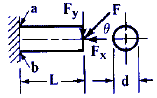Circular Cantilever Beam in Direct Compression and Bending Equations and Calculator

Circular Cantilever Beam in Direct Compression and Bending Equations and CalculatorRule 1: For every calculated normal stress there is a corresponding induced shear stress; the value of the shear stress is equal to half that of the normal stress.

Rule 2: For every calculated shear stress there is a corresponding induced normal stress; the value of the normal stress is equal to that of the shear stress. The tables of combined stress formulas, below, include equations for calculating both maximum nominal tensile or compressive stresses, and maximum nominal shear stresses.

Maximum Nominal Tensile or Compressive StressMaximum Nominal
Shear StressWhere:

 d = Diameter inches (mm) L = Length of Beam inches (mm) Fy = Applied Load lbs (N) Fx = Applied Load lbs (N) σ' a = Stress, Tensile or Compressive Lbs/in2 (N/mm2) σ' b = Stress, Tensile or Compressive Lbs/in2 (N/mm2) τ'a = Stress , Max. Nominal Shear Lbs/in2 (N/mm2) τ'b = Stress , Max. Nominal Shear Lbs/in2 (N/mm2)

References:

1. Any Machinery's Handbook published since 1931 or,
2. Machinery's Handbook, 21st Edition, Page 404 or,
3. Machinery's Handbook, 23st Edition, Page 260 or,
4. Machinery's Handbook, 27st Edition, Page 261Membership Register | LoginHomeEngineering Book StoreEngineering ForumExcel App. DownloadsOnline Books & ManualsEngineering NewsEngineering VideosEngineering CalculatorsEngineering ToolboxGD&T Training Geometric Dimensioning TolerancingDFM DFA TrainingTraining Online EngineeringAdvertising CenterCopyright Notice